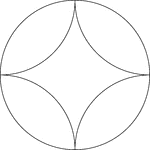### Arcs Inscribed In A Circle

A design created by inscribing 4 congruent tangent arcs in a circle.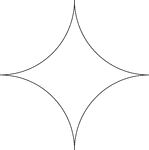### Reflected Arcs Of A Circle

A design created by dividing a circle into 4 equal arcs and reflecting each arc toward the center of…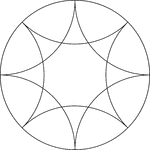### Reflected Arcs Of 2 Circles In A Circle

A design created by dividing a circle into 4 equal arcs and creating a reflection of each arc toward…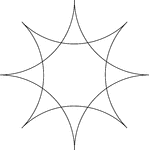### Reflected Arcs Of 2 Circles

A design created by dividing a circle into 4 equal arcs and reflecting each arc toward the center of…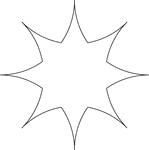### Reflected Arcs Of 2 Circles

A design created by dividing a circle into 4 equal arcs and reflecting each arc toward the center of…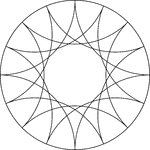### Reflected Arcs Of 4 Circles In A Circle

A design created by dividing a circle into 4 equal arcs and creating a reflection of each arc toward…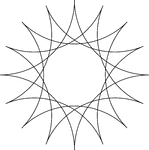### Reflected Arcs Of 4 Circles In A Circle

A design created by dividing a circle into 4 equal arcs and creating a reflection of each arc toward…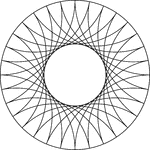### Reflected Arcs Of 8 Circles In A Circle

A design created by dividing a circle into 4 equal arcs and creating a reflection of each arc toward…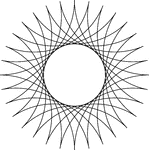### Reflected Arcs Of 8 Circles

A design created by dividing a circle into 4 equal arcs and creating a reflection of each arc toward…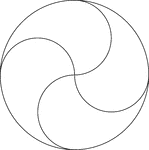### Circular Design

Circular design made by rotating circles about a fixed point. The radii of the smaller circles is equal…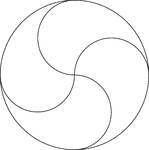### Circular Design

Circular design made by rotating circles about a fixed point. The radii of the smaller circles is equal…